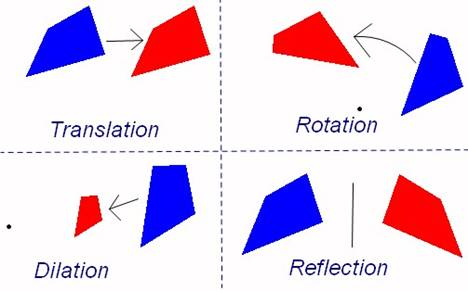# Location and Movement

##BIG IDEAS:

(taken from “Big Ideas by Dr. Small”):

1. Locations can be described using maps, using length and angle measurements, and using coordinate grids.
2. Congruent shapes have identical side lengths, angles, perimeters, and areas.
3. Similar shapes have identical angles and are the same shape but are a different size.
4. There are three motions, or transformations, that change the position of a shape or possibly its orientation, but do not change its size and shape (translations, reflections, and rotations). Other transformations (like dilatations) can affect size.
5. Transformations are frequently observable in our everyday world. One example context is a tessellation.

## STUDENT LEARNING GOALS:

GOAL #1: I can plot and identify points in all quadrants on the Cartesian plane.

GOAL #2: I can identify congruent shapes and justify why certain triangles are considered congruent.

GOAL #3: I can identify similar shapes and perform dilatations.

GOAL #4: I can identify and perform transformations and tessellations.

## CURRICULUM EXPECTATIONS:

• Develop an understanding of similarity, and distinguish similarity and congruence;
• Plot points using all four quadrants of the Cartesian coordinate plane;
• Describe location in the four quadrants of a coordinate system, dilatate two-dimensional shapes, and apply transformations to create and analyse designs;
• Identify, through investigation, the minimum side and angle information (i.e., side-side-side; side-angle-side; angle-side-angle) needed to describe a unique triangle (e.g., “I can draw many triangles if I’m only told the length of one side, but there’s only one triangle I can draw if you tell me the lengths of all three sides.”);
• Determine, through investigation using a variety of tools (e.g., dynamic geometry software, concrete materials, geoboard), relationships among area, perimeter, corresponding side lengths, and corresponding angles of congruent shapes;
• Demonstrate an understanding that enlarging or reducing two-dimensional shapes creates similar shapes;
• Distinguish between and compare similar shapes and congruent shapes, using a variety of tools (e.g., pattern blocks, grid paper, dynamic geometry software) and strategies (e.g., by showing that dilatations create similar shapes and that translations, rotations, and reflections generate congruent shapes).
• Identify, perform, and describe dilatations (i.e., enlargements and reductions), through investigation using a variety of tools (e.g., dynamic geometry software, geoboard, pattern blocks, grid paper);
• Create and analyse designs involving translations, reflections, dilatations, and/or simple rotations of two-dimensional shapes, using a variety of tools (e.g., concrete materials, Mira, drawings, dynamic geometry software) and strategies (e.g., paper folding);
• Determine, through investigation using a variety of tools (e.g., pattern blocks, Polydrons, grid paper, tiling software, dynamic geometry software, concrete materials), polygons or combinations of polygons that tile a plane, and describe the transformation(s) involved.